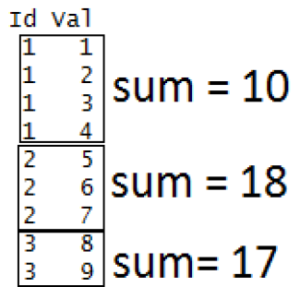GFG App
Open AppBrowser
Continue

# Looping over Objects in R Programming

Prerequisite: Data Structures in R Programming

One of the biggest issues with the “for” loop is its memory consumption and its slowness in executing a repetitive task. And when it comes to dealing with large data set and iterating over it, for loop is not advised. R provides many alternatives to be applied to vectors for looping operations that are pretty useful when working interactively on a command line. In this article, we deal with `apply()` function and its variants:

``` apply() lapply() sapply() tapply() mapply() ```

Let us see what each of these functions does.

Looping Function Operation
`apply()` Applies a function over the margins of an array or matrix
`lapply()` Apply a function over a list or a vector
`sapply()` Same as `lapply()` but with simplified results
`tapply()` Apply a function over a ragged array
`mapply()` Multivariate version of `lapply()`
• `apply()`: This function applies a given function over the margins of a given array.

apply(array, margins, function, …)

array = list of elements
margins = dimension of the array along which the function needs to be applied
function = the operation which you want to perform

Example:

 `# R program to illustrate ` `# apply() function ` ` `  `# Creating a matrix ` `A = ``matrix``(1:9, 3, 3) ` `print``(A) ` ` `  `# Applying apply() over row of matrix ` `# Here margin 1 is for row  ` `r = ``apply``(A, 1, sum) ` `print``(r) ` ` `  `# Applying apply() over column of matrix ` `# Here margin 2 is for column ` `c = ``apply``(A, 2, sum) ` `print``(c) `

Output:

```[, 1] [, 2] [, 3]
[1, ]    1    4    7
[2, ]    2    5    8
[3, ]    3    6    9

 12 15 18
  6 15 24
```
• `lapply():` This function is used to apply a function over a list. It always returns a list of the same length as the input list.

lapply(list, function, …)

list = Created list
function = the operation which you want to perform

Example:

 `# R program to illustrate ` `# lapply() function ` ` `  `# Creating a matrix ` `A = ``matrix``(1:9, 3, 3) ` ` `  `# Creating another matrix ` `B = ``matrix``(10:18, 3, 3)  ` ` `  `# Creating a list ` `myList = ``list``(A, B) ` ` `  `# applying lapply() ` `determinant = ``lapply``(myList, det) ` `print``(determinant) `

Output:

```[]
 0

[]
 5.329071e-15
```
• `sapply():` This function is used to simplify the result of `lapply()`, if possible. Unlike `lapply()`, the result is not always a list. The output varies in the following ways:-
• If output is a list containing elements having length 1, then a vector is returned.
• If output is a list where all the elements are vectors of same length(>1), then a matrix is returned.
• If output contains elements which cannot be simplified or elements of different types, a list is returned.

sapply(list, function, …)

list = Created list
function = the operation which you want to perform

Example:

 `# R program to illustrate ` `# sapply() function ` ` `  `# Creating a list ` `A = ``list``(a = 1:5, b = 6:10) ` ` `  `# applying sapply() ` `means = ``sapply``(A, mean) ` `print``(means) `

Output:

```a b
3 8
```

A vector is returned since the output had a list with elements of length 1.

• `tapply()`: This function is used to apply a function over subset of vectors given by a combination of factors.

tapply(vector, factor, function, …)

vector = Created vector
factor = Created factor
function = the operation which you want to perform

Example:

 `# R program to illustrate ` `# tapply() function ` ` `  `# Creating a factor ` `Id = ``c``(1, 1, 1, 1, 2, 2, 2, 3, 3) ` ` `  `# Creating a vector ` `val = ``c``(1, 2, 3, 4, 5, 6, 7, 8, 9) ` ` `  `# applying tapply() ` `result = ``tapply``(val, Id, sum) ` `print``(result) `

Output:

```1  2  3
10 18 17
```

How does the above code work?• `mapply()`: It’s a multivariate version of `lapply()`. This function can be applied over several list simultaneously.

mapply(function, list1, list2, …)

function = the operation which you want to perform

list1, list2 = Created lists

Example:

 `# R program to illustrate ` `# mapply() function ` ` `  `# Creating a list ` `A = ``list``(``c``(1, 2, 3, 4)) ` ` `  `# Creating another list ` `B = ``list``(``c``(2, 5, 1, 6)) ` ` `  `# Applying mapply() ` `result = ``mapply``(sum, A, B) ` `print``(result) `

Output:

``` 24
```

My Personal Notes arrow_drop_up